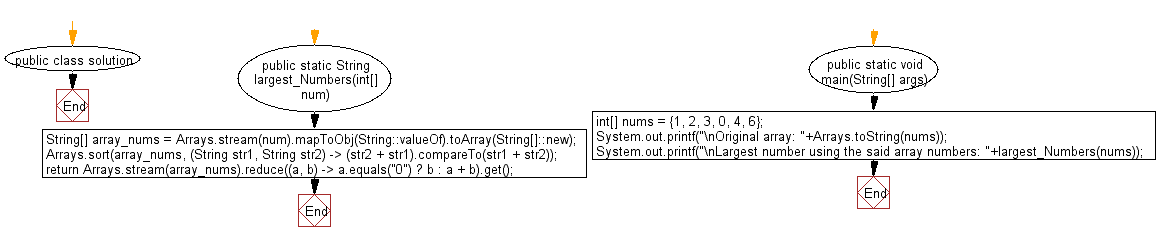﻿ Java: Largest number from a list of non negative integers# Java Array Exercises: Form the largest number from a given list of non negative integers

## Java Array: Exercise-71 with Solution

Write a Java program to form the largest number from a given list of non negative integers.

Example:
Input :
nums = {1, 2, 3, 0, 4, 6}
Output:
Largest number using the said array numbers: 643210

Sample Solution:

Java Code:

``````import java.util.*;
public class solution {

public static String  largest_Numbers(int[] num) {
String[] array_nums = Arrays.stream(num).mapToObj(String::valueOf).toArray(String[]::new);
Arrays.sort(array_nums, (String str1, String str2) -> (str2 + str1).compareTo(str1 + str2));
return Arrays.stream(array_nums).reduce((a, b) -> a.equals("0") ? b : a + b).get();
}

public static void main(String[] args)
{
int[] nums = {1, 2, 3, 0, 4, 6};
System.out.printf("\nOriginal array: "+Arrays.toString(nums));
System.out.printf("\nLargest number using the said array numbers: "+largest_Numbers(nums));
}
}
```
```

Sample Output:

```Original array: [1, 2, 3, 0, 4, 6]
Largest number using the said array numbers: 643210
```

Flowchart:Java Code Editor:

Improve this sample solution and post your code through Disqus

What is the difficulty level of this exercise?

Test your Programming skills with w3resource's quiz.

﻿

## Java: Tips of the Day

countOccurrences

Counts the occurrences of a value in an array.

Use Arrays.stream().filter().count() to count total number of values that equals the specified value.

```public static long countOccurrences(int[] numbers, int value) {
return Arrays.stream(numbers)
.filter(number -> number == value)
.count();
}
```

Ref: https://bit.ly/3kCAgLb